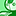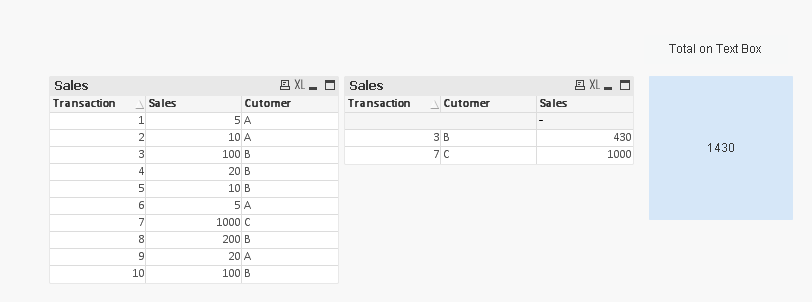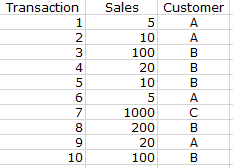# QlikView App Dev

Discussion Board for collaboration related to QlikView App Development.

Announcements
Join this live chat April 6, 10AM EST - QlikView to Qlik Sense REGISTER
cancel
Showing results for
Did you mean:Not applicable

## Sum of an aggregation if the aggregation meets a calculated threshold

Hi Community,

I am trying to get the sum sales of all customers that have total sales above a certain level. The issue I'm running into is that the data is at a transactional level, so the formula must first aggregate sales at the customer level and then assess those aggregated values.

I have tried the following without luck:

IF(

AGGR(SUM(Sales), Customer) >= v.CUSTFRACTILE,

SUM(SALES))

and

IF(

AGGR(SUM(Sales), Customer) >= v.CUSTFRACTILE,

SUM(AGGR(SUM(Sales), Customer)))

Any help would be greatly appreciated!

Thank you,

1 Solution

Accepted SolutionsContributor III

Something like this ?Regards!

9 RepliesChampion III

Can you share some sample data with an expected output.Not applicable
Author

Hi Vishwarath,

I can't share the actual data but I have created a simple example below:If in this example I'm looking for the sum of sales for all customers that have sales of at least 400, the correct answer would be 1430 (sum of sales for customers B and C since they spent 430 and 1000 respectively).Champion III

Are you looking for this?Assuming that you already have set the variable vCustfractile to 400.

Then in your straight table add Customer and Transaction and expression as

= IF(Sum(Aggr(Sum(SalesAmt), Customer)) >= vCustfractile, Sum(Aggr(Sum(SalesAmt), Customer)))

With Total Mode as Sum of Rows Radio check.Not applicable
Author

Thanks, Vishwarath.

I'm looking to get the number to display in a text  box since I'm using it as a KPI at the top of a dashboard. Is there a way of calculating this without using a straight table (or alternately referencing a straight table total from a text box)?Contributor III

Something like this ?Regards!Not applicable
Author

The SUM(IF( statement works perfectly - Thank you!Creator II

How to get this 1430 in a text box using set expression?  assuming we have vCUSTFRACTILE as variable.MVP

May be this

=Sum({<Cutomer = {"=Sum(Sales) > \$(vCUSTFRACTILE)"}>}Sales)Creator II

Perfect thanks Much Sunny.